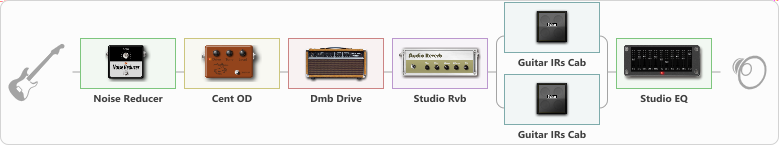# Crunch

Discussion in 'ToneLib-GFX presets' started by Dzmitry, Apr 19, 2021.

1. Crunch

Preset name: MY Crunch

Effects chain:Effect: "Noise Reducer" (Динамика / Фильтр), active - "yes"
{
"Sens" = 73
"Mode" = Soft
}

Effect: "Cent OD" (Перегрузка / Искажение), active - "yes"
{
"Drive" = 50
"Tone" = 70
"Level" = 100
}

Effect: "Dmb Drive" (Усилители симуляторы), active - "yes"
{
"Gain" = 50
"Bass" = 53
"Middle" = 25
"Treble" = 54
"Presence" = 80
"Master" = 100
"Level (dB)" = 0
}

Effect: "Studio Rvb" (Реверберация), active - "yes"
{
"Time" = 5.4
"PreDelay" = 30
"LoDamp" = 0
"HiDamp" = 12
"Mix" = 48
}

Effect: "Splitter" (Динамика / Фильтр)
{
"A-Bypass" = Off
"A-Pan" = 0
"A-Level" = 55
"B-Bypass" = Off
"B-Pan" = 0
"B-Level" = 60

'A' branch:
{

Effect: "Guitar IRs Cab" (Кабинеты), active - "yes"
{
"Model" = Marshall 1960A (4x12")
"Mic Position" = Middle
"Mic Distance" = Far
"Low Cut (Hz)" = 80
"Hi Cut (kHz)" = 10.0
"Mix" = 100
"Level (dB)" = 0
}
}
'B' branch:
{

Effect: "Guitar IRs Cab" (Кабинеты), active - "yes"
{
"Model" = Soldano Slant (4x12")
"Mic Position" = Center
"Mic Distance" = Middle
"Low Cut (Hz)" = 80
"Hi Cut (kHz)" = 20.0
"Mix" = 100
"Level (dB)" = 0
}
}
}

Effect: "Studio EQ" (Динамика / Фильтр), active - "yes"
{
"31 Hz" = -15
"62 Hz" = -4
"125 Hz" = -2
"250 Hz" = -1
"500 Hz" = -7
"1 kHz" = -3
"2 kHz" = -1
"4 kHz" = -5
"8 kHz" = 2
"16 kHz" = 0
"Level (dB)" = 0
}

Note: You will need to download and install the ToneLib-GFX software to use the preset.

#### Attached Files:

• ###### Crunch.tlgfx
File size:
832 bytes
Views:
2,311
Sergejs Matvejenkovs likes this.